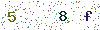﻿ Have a Look at Apparent power - LISUNLISUN

# Have a Look at Apparent power

Apparent power is the product of the ac voltage and ac current. Expressed in formula is: S = UI. In the formula, S is the rated power output, the unit is VA (ei); U is rated output voltage, the unit is V, such as 220 V, 220 V, etc.; I was rated output current, the unit is A. In fact, apparent power includes two parts: active power (P) and reactive power (Q). Active power refers to the direct work part which makes lights shine, the motor rotate, the electronic circuit work, etc. As a result, the power turned into heat after working and can be felt by people directly, and some people will produce an illusion, regarding the active power as the apparent power, but does not know active power is only part of the apparent power, expressed in formula: P＝Scosθ＝UIcosθ＝UI*F. Type, in this formula, P is the active power, the unit is W (watt); F＝cosθ is called power factor, and the θ is the phase difference in different voltage and current phase with nonlinear loads. Reactive power is part of the power that stored in the circuit but not directly to work.